## Resource of the Week: probability as a fraction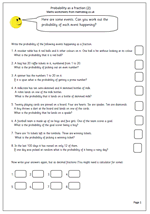Probability does cause some confusion with children, partly because it can be expressed in several ways and because it is very hard to find events which are absolutely certain to happen, or not happen.

One way of displaying the probability of an event is as a percentage: for example, there is a 50% chance of landing on a head when tossing a coin.

Another way is as a fraction: there is 1/2 chance, or one in two chance of landing on heads.

A third way is as a decimal fraction, where zero means no chance and 1 is certain: there is 0.5 chance of landing on heads. Probability can be displayed along a number line marked from zero to one.

A the end of the page there is a tricky probability line where the events have to be placed in order of likelihood.

This year 6 maths worksheet is the second published which looks at probability as a fraction and then as a decimal fraction. A calculator would be useful to do the conversion and it is suggested that the answer should be displayed to 2 decimal places. A useful homework sheet.

Giving the probability as a fraction_(pg 2)

## Year 3 maths worksheet: adding three numbers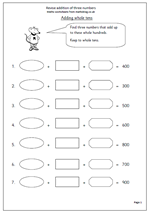Here we have a simple page which looks at adding three multiples of ten to make a variety of whole hundreds. It is quite open ended as there are many possible answers and the page could be repeated several times or used as the starting point for a mini investigation.

The page could be answerred very simply eg 280 + 10 + 10 = 300

but encourage children to make it as tricky as possible to show how good they are at maths;

e.g. 30 + 120 + 150.

This page can be found in our Year 3 Calculations category, together with many others.

## Standard written method for adding decimals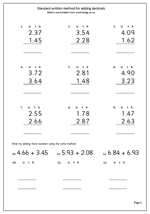Here we have a very straightforward page of addition questions with decimals. All the numbers use 2 decimal places, so it is very similar to adding money. I have also kept to adding just two numbers so this page is ideal for children who are getting to grips with the standard method of addition.

The only real cause for problems is the decimal point and it is vital that this is written in the answer in line with the other decimal points. The first 9 questions have already been laid out in the correct way but the last three need to rewritten.

Later children will need to add numbers where the number of digits after the decimal point varies eg. 26.4 + 1.78 and it will help enormously if the are confident with how to set them out.

The speed of answering this type of question depends on how well number bonds (eg 5 + 6) have been learned ‘off by heart’.

## Useful resources: 1 to 100 number grid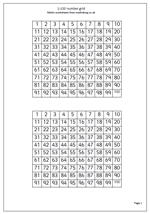Having a browse round the site I noticed that there is not very much in the Useful Resources section so I thought it was about time I added some! A number grid (often called a number square) from 1-100 is a very useful resource, especially when used for counting and looking for patterns. An obvious use is to help children count up in ones to 100, but it can also be used for more complex counting.

For example, counting in threes and colouring each third number will give an interesting diagonal whilst counting in fives will produce two vertical lines. Do them both on the same grid will show which numbers are divisible by both 3 and 5 e.g. 15 and 30.

Number grids such as this can also be used as an aid for addition and subtraction.

Go to 1-100 number grid

## Calculating in Year 2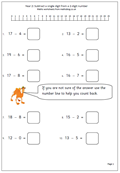In Year 2 children will be calculating using the addition, subtraction, multiplication and division symbols. Their understanding of addition will develop as they begin to add a 1-digit number to a 2-digit number as well as beginning to answer written addition questions. (This is not the same as using written methods.) Developing efficient mental methods of addition and subtraction are still the most important aspects here, as written methods, apart from jottings, should not yet be used.

Subtraction is much easier once it is realised that it is the inverse (or opposite) of addition. Many subtraction questions can be answered by adding on, but children are expected to know subtraction facts to at least 10 (eg know that 9 – 4 = 5). Knowing these facts is just as important as knowing ‘tables’. They will also be working with 2-digit numbers, especially multiples of 10.

There is a good selection of addition and subtraction worksheets on the site in our Year 2 calculating section as well as a growing number of multiplication and division pages. Multiplication is seen as repeated addition and the 2x, 5x and 10x tables will be learned during the year.

As well as in the Calculating section further worksheets can be found in Knowing Number Facts and our Four Rules section.

Go to Year 2 calculating worksheets

## Divide 3-digit numbers by 6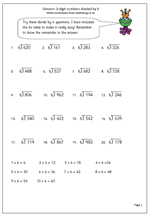This is a short division page which concentrates just on dividing 3-digit numbers by 6. Short division, as its name suggests is a shortened version of the long division method where subtractions and remainders are done mentally.

The key to answering these is to have a set speech for the process.

For example: 6)457

‘How many sixes in 45?’

‘7 times 6 is 42.’

‘6 goes into 45, 7 times with 3 left over.’

Write the 7 in the answer above the 5.

Write the remainder three beside the 7 units, making 37.

‘How many sixes in 37?’

‘6 times 6 is 36.’

‘6 goes into 37, 6 times with 1 left over.’

Write the 6 in the answer in the units and write rem 1 next to it.

Division: 3-digit numbers by 6

## Resource of the Week: Bar charts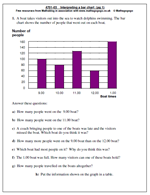Now we are coming on to more familiar territory for most people when we think of graphs: the bar chart or bar graph. Here the vertical bars represent the number of people going out on a boat. Each bar is completely separate from the others.

It is important that children get used to naming the horizontal and vertical axes and that these axes should always be named.

The hardest part of interpreting this bar chart involves the number of people shown on the vertical axis.The numbers go up in twenties, but of course there are intermediate values. The number of people on the 11.00 boat is more difficult to read and only a close approximation between 120 and 140 can be made. As it looks just less than half way to the next interval an estimate of just below 130 would be very accurate.

It is important not to just look at the graph but to also make some suggestions as to why the results are as they are. Why did the 12.00 boat have the least number of people on it, but the 1.00 boat had the most?

The second worksheet has similar problems, but the numbers on the vertical axis go up in tens rather than twenties.

Free Y4 maths worksheet: Bar charts (pg 1)

Free Y4 maths worksheet: Bar charts (pg 2)

## Counting and Number in year 2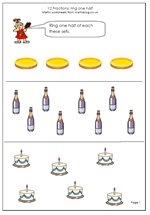A great deal is expected of children by the end of Year 2 and we have some great maths worksheets to help them on their way.

By the end of the year children are expected to read and write 2-digit numbers in figures and words, describe and extend number sequences and recognise odd and even numbers.

They should continue to gain confidence with counting in singe steps up to at least 100 and begin to count in twos, fives and tens. Estimating a number of objects and rounding 2-digit numbers to the nearest ten are also introduced in Year 2.

There are a number of pages on counting on and back in ones and tens, finding missing numbers on number squares as well as recognising simple fractions.

Go to our year 2 Counting and Number worksheets

## Short multiplication (multiplying by 3)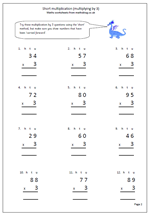Today I have published a page of multiplication questions which can be used to reinforce knowledge of the 3 times table as well as practising the short method of multiplication. it is called the short method because it does not involve writing to answer to each part of the question on a new line and then adding the totals, rather it is all done on one line. The main thing to remember is to carry the extra tens into the tens column and remember to add them into the final answer. A page at the start shows how to go about using this method.

The primary Framework for Mathematics is very keen to emphasise that written methods of multiplication should only be used when children have established a sound foundation of mental methods.

This page can be found in the Four Rules, Multiplication category, along with lots more.

Short multiplication for multiplying by 3

## Resource of the Week: Completing number squares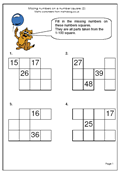This week we are highlighting a Year 2 worksheet on number squares. This is our second in a series for year 2 children where they have to complete the missing numbers in a 100 square. Some of the squares have been removed from the rectangles which, curiously, seems to make this task harder than when working with a complete rectangle. This is good practice for children who are not confident with counting up to 100. Don’t be surprised if children stumble over counting up to 100 out loud. It is something we often take for granted, but of course, has to be learned and many children find it quite difficult, especially when crossing the tens boundaries (e.g. from 79 to 80).

Thanks to URBrainy for letting me use this page. They are by no means the best site around for Key Stage 1 and you can try it out for nothing here.

Missing numbers on a number (p 2)